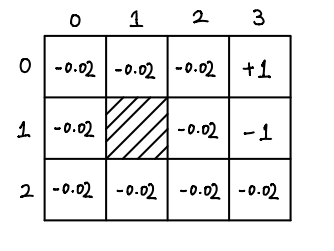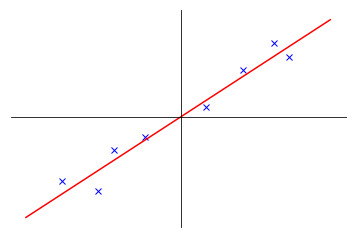# Markov Decision Process: Finite horizon

This post is considered to the notes on finite horizon Markov decision process for lecture 18 in Andrew Ng's lecture series. In my previous two notes (, ) about Markov decision process (MDP), only state rewards are considered. We can easily generalize MDP to state-action reward.

## State-Action Reward

Our reward function now is a function in terms of both states and actions. More precisely, the reward function is a function

$$R: S \times A \to \R.$$

All other requirements in definition of MDP will remain intact. For completeness, we include the definition here. We shall pay attention to the fifth components.

# Markov Decision Process: Continuous states

I wrote this post for lecture 17 in Andrew Ng's lecture collections on Machine Learning. In my previous post, we discussed Markov Decision Process (MDP) in its simplest form, where the set of states and the set of actions are both finite. But in real world application, states and actions can be infinite and even continuous. For example, if we want to model states of a self-driving car in a 2D plane, we must at least have the position $(x, y)$, the direction $\theta$ of the car pointing to, its velocity $(v_x, v_y)$ and the rate $r$ of change in $\theta$. So the states of a car is at least a 6 dimensional space. For actions of a car, we can control how fast it goes in direction $\theta$ and we can also control $r$. Thus the actions have dimension 2.

In this post, we consider only continuous states with finite actions. Indeed, actions space usually has much lower dimension than states space, so in case of continuous actions, we might just discretize the actions spaces to get a finite set of representatives of actions. One may argue that we can also discretize the states space. Yes, we can do it, but only when the dimension $n$ of state space is small enough: if we discretize each dimension into $k$ parts, then there would be $k^n$ many states. If $n$ is large, then $k^n$ is not feasible. This is so called the curse of dimensionality. Moreover, discretizing states space usually results in lack of smoothness.

# Markov Decision Process

This article is my notes for 16th lecture in Machine Learning by Andrew Ng on Markov Decision Process (MDP). MDP is a typical way in machine learning to formulate reinforcement learning, whose tasks roughly speaking are to train agents to take actions in order to get maximal rewards in some settings. One example of reinforcement learning would be developing a game bot to play Super Mario on its own.

Another simple example is used in the lecture, and I will use it throughout the post as well. Since the example is really simple, so MDP shown below is not of the most general form, but only good enough to solve the example and give the idea of what MDP and reinforcement learning are. The example begins with a 3 by 4 grid as below.# Principal Component Analysis

This article is my notes on Principal Component Analysis (PCA) for Lecture 14 and 15 of Machine Learning by Andrew Ng. Given a set of high dimensional data $\{x^{(1)}, \dots, x^{(m)}\}$, where each $x^{(i)} \in \R^{n}$, with the assumption that these data actually roughly lie in a much smaller $k$ dimensional subspace, PCA tries to find a basis for this $k$ dimensional subspace. Let's look at a simple example:# 烤猪排# LeetCode Contest 60

class Solution(object):
def floodFill(self, image, sr, sc, newColor):
"""
:type image: List[List[int]]
:type sr: int
:type sc: int
:type newColor: int
:rtype: List[List[int]]
"""
visited = set()
n = len(image)
m = len(image)
color = image[sr][sc]

def dfs(r, c):
image[r][c] = newColor
for dr, dc in [(0, 1), (1, 0), (0, -1), (-1, 0)]:
x, y = r+dr, c+dc
if x < 0 or y < 0 or x >= n or y >= m or image[x][y] != color:
continue
if (x, y) in visited:
continue
dfs(x, y)

dfs(sr, sc)
return image


# 蒜蓉鸡蛋面# Factor Analysis

This article is my notes on the topic of factor analysis. These notes come out of lecture 13 and 14 of Andrew Ng's online course. Roughly speaking, factor analysis models some $n$ dimensional observed data with the assumption that these data are actually from some $d$ dimensional plane in $\R$, up to some Gaussian distributed errors. Let's make it more precise.

Suppose we have a set of observed data $\{x^{(1)}, \dots, x^{(m)}\}$ implicitly labeled by some latent random variable $z \in \R^d$ where

$$z \sim \mathcal{N}(0, I).$$

Factor analysis model tries to model $P(x)$ using the assumption that

$$\begin{equation} x|z \sim \mathcal{N}(\mu+\Lambda z, \Psi), \label{cond-xz} \end{equation}$$

for some $\mu \in \R^n, \Lambda \in \R^{n \times d}$ and diagonal matrix $\Psi \in \R^{n \times n}$. These $\mu, \Lambda$ and $\Psi$ are parameters of the model.

# Expectation-Maximization algorithm

In this article, I will collect my notes on Expectation-Maximization algorithm (EM) based on lecture 12 and 13 of Andrew Ng's online course. Given a set of unlabeled data points EM tries iteratively to determine the distribution of data, assuming that all data points are implicitly labeled (unobserved latent variables). For simplicity, we shall limit ourselves to the case where there are only finitely many implicit labels.

## Description of the problem

Given a set of unlabeled data $\{x^{(1)}, \dots, x^{(m)}\}$, our goal is to determine $P(x)$, the distribution of $x$, with the following assumptions.

Assumptions.

1. There are finitely many unobserved latent variables $z \in \{1, \dots, k\}$ and they obey some multinomial distribution, i.e., $P(z=j) = \phi_j$ with $\sum \phi_j = 1$.

2. $\{P(x|z=j; a_j): j=1, \dots, k\}$ are a family of uniformly parametrized distribution.

Assumptions 1 and 2 will gives us a set of parameters $\theta = (\phi_1, \dots, \phi_j, a_1,\dots, a_j)$ and

$$\begin{equation} P(x; \theta) = \sum_{j=1}^k P(x|z=j; \theta)P(z=j; \theta). \label{px} \end{equation}$$

We want to find this set of parameters so that the likelihood function

$$L(\theta) = \prod_{i=1}^m P(x^{(i)}) = \prod_{i=1}^m \sum_{j=1}^k P(x^{(i)}|z=j; \theta)P(z=j; \theta).$$

is maximized. Or equivalently, the log likelihood function below is maximized:

$$\begin{equation} l(\theta) = \sum_{i=1}^m \log\left(\sum_{j=1}^k P(x^{(i)}, z=j; \theta)\right), \label{log-likelihood} \end{equation}$$

where

$$P(x^{(i)}, z=j; \theta) = P(x^{(i)}|z=j; \theta)P(z=j; \theta).$$